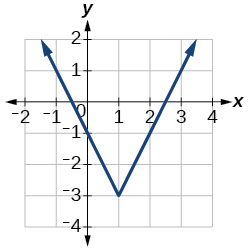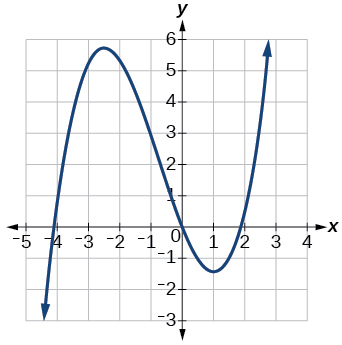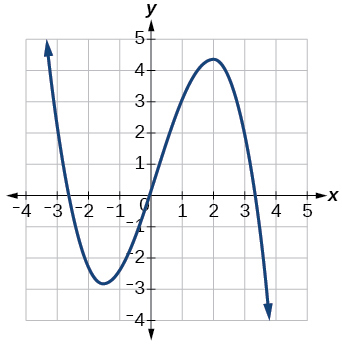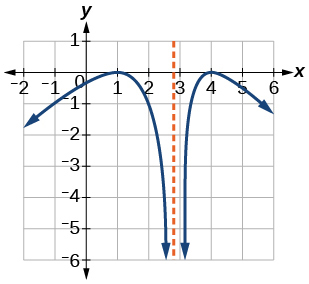3.3 Rates of change and behavior of graphs  (Page 5/15)

 Page 5 / 15

Access this online resource for additional instruction and practice with rates of change.

Key equations

 Average rate of change $\frac{\Delta y}{\Delta x}=\frac{f\left({x}_{2}\right)-f\left({x}_{1}\right)}{{x}_{2}-{x}_{1}}$

Key concepts

• A rate of change relates a change in an output quantity to a change in an input quantity. The average rate of change is determined using only the beginning and ending data. See [link] .
• Identifying points that mark the interval on a graph can be used to find the average rate of change. See [link] .
• Comparing pairs of input and output values in a table can also be used to find the average rate of change. See [link] .
• An average rate of change can also be computed by determining the function values at the endpoints of an interval described by a formula. See [link] and [link] .
• The average rate of change can sometimes be determined as an expression. See [link] .
• A function is increasing where its rate of change is positive and decreasing where its rate of change is negative. See [link] .
• A local maximum is where a function changes from increasing to decreasing and has an output value larger (more positive or less negative) than output values at neighboring input values.
• A local minimum is where the function changes from decreasing to increasing (as the input increases) and has an output value smaller (more negative or less positive) than output values at neighboring input values.
• Minima and maxima are also called extrema.
• We can find local extrema from a graph. See [link] and [link] .
• The highest and lowest points on a graph indicate the maxima and minima. See [link] .

Verbal

Can the average rate of change of a function be constant?

Yes, the average rate of change of all linear functions is constant.

If a function $\text{\hspace{0.17em}}f\text{\hspace{0.17em}}$ is increasing on $\text{\hspace{0.17em}}\left(a,b\right)\text{\hspace{0.17em}}$ and decreasing on $\text{\hspace{0.17em}}\left(b,c\right),\text{\hspace{0.17em}}$ then what can be said about the local extremum of $\text{\hspace{0.17em}}f\text{\hspace{0.17em}}$ on $\text{\hspace{0.17em}}\left(a,c\right)?\text{\hspace{0.17em}}$

How are the absolute maximum and minimum similar to and different from the local extrema?

The absolute maximum and minimum relate to the entire graph, whereas the local extrema relate only to a specific region around an open interval.

How does the graph of the absolute value function compare to the graph of the quadratic function, $\text{\hspace{0.17em}}y={x}^{2},\text{\hspace{0.17em}}$ in terms of increasing and decreasing intervals?

Algebraic

For the following exercises, find the average rate of change of each function on the interval specified for real numbers $\text{\hspace{0.17em}}b\text{\hspace{0.17em}}$ or $\text{\hspace{0.17em}}h$ in simplest form.

$f\left(x\right)=4{x}^{2}-7\text{\hspace{0.17em}}$ on

$4\left(b+1\right)$

$g\left(x\right)=2{x}^{2}-9\text{\hspace{0.17em}}$ on

$p\left(x\right)=3x+4\text{\hspace{0.17em}}$ on

3

$k\left(x\right)=4x-2\text{\hspace{0.17em}}$ on

$f\left(x\right)=2{x}^{2}+1\text{\hspace{0.17em}}$ on $\text{\hspace{0.17em}}\left[x,x+h\right]$

$4x+2h$

$g\left(x\right)=3{x}^{2}-2\text{\hspace{0.17em}}$ on $\text{\hspace{0.17em}}\left[x,x+h\right]$

$a\left(t\right)=\frac{1}{t+4}\text{\hspace{0.17em}}$ on $\text{\hspace{0.17em}}\left[9,9+h\right]$

$\frac{-1}{13\left(13+h\right)}$

$b\left(x\right)=\frac{1}{x+3}\text{\hspace{0.17em}}$ on $\text{\hspace{0.17em}}\left[1,1+h\right]$

$j\left(x\right)=3{x}^{3}\text{\hspace{0.17em}}$ on $\text{\hspace{0.17em}}\left[1,1+h\right]$

$3{h}^{2}+9h+9$

$r\left(t\right)=4{t}^{3}\text{\hspace{0.17em}}$ on $\text{\hspace{0.17em}}\left[2,2+h\right]$

$\frac{f\left(x+h\right)-f\left(x\right)}{h}\text{\hspace{0.17em}}$ given $\text{\hspace{0.17em}}f\left(x\right)=2{x}^{2}-3x\text{\hspace{0.17em}}$ on $\text{\hspace{0.17em}}\left[x,x+h\right]$

$4x+2h-3$

Graphical

For the following exercises, consider the graph of $\text{\hspace{0.17em}}f\text{\hspace{0.17em}}$ shown in [link] .

Estimate the average rate of change from $\text{\hspace{0.17em}}x=1\text{\hspace{0.17em}}$ to $\text{\hspace{0.17em}}x=4.$

Estimate the average rate of change from $\text{\hspace{0.17em}}x=2\text{\hspace{0.17em}}$ to $\text{\hspace{0.17em}}x=5.$

$\frac{4}{3}$

For the following exercises, use the graph of each function to estimate the intervals on which the function is increasing or decreasing.increasing on $\text{\hspace{0.17em}}\left(-\infty ,-2.5\right)\cup \left(1,\infty \right),\text{\hspace{0.17em}}$ decreasing onincreasing on $\text{\hspace{0.17em}}\left(-\infty ,1\right)\cup \left(3,4\right),\text{\hspace{0.17em}}$ decreasing on $\text{\hspace{0.17em}}\left(1,3\right)\cup \left(4,\infty \right)$

For the following exercises, consider the graph shown in [link] .

A laser rangefinder is locked on a comet approaching Earth. The distance g(x), in kilometers, of the comet after x days, for x in the interval 0 to 30 days, is given by g(x)=250,000csc(π30x). Graph g(x) on the interval [0, 35]. Evaluate g(5)  and interpret the information. What is the minimum distance between the comet and Earth? When does this occur? To which constant in the equation does this correspond? Find and discuss the meaning of any vertical asymptotes.
The sequence is {1,-1,1-1.....} has
how can we solve this problem
Sin(A+B) = sinBcosA+cosBsinA
Prove it
Eseka
Eseka
hi
Joel
June needs 45 gallons of punch. 2 different coolers. Bigger cooler is 5 times as large as smaller cooler. How many gallons in each cooler?
7.5 and 37.5
Nando
find the sum of 28th term of the AP 3+10+17+---------
I think you should say "28 terms" instead of "28th term"
Vedant
the 28th term is 175
Nando
192
Kenneth
if sequence sn is a such that sn>0 for all n and lim sn=0than prove that lim (s1 s2............ sn) ke hole power n =n
write down the polynomial function with root 1/3,2,-3 with solution
if A and B are subspaces of V prove that (A+B)/B=A/(A-B)
write down the value of each of the following in surd form a)cos(-65°) b)sin(-180°)c)tan(225°)d)tan(135°)
Prove that (sinA/1-cosA - 1-cosA/sinA) (cosA/1-sinA - 1-sinA/cosA) = 4
what is the answer to dividing negative index
In a triangle ABC prove that. (b+c)cosA+(c+a)cosB+(a+b)cisC=a+b+c.
give me the waec 2019 questionsByByBy Nick Swain